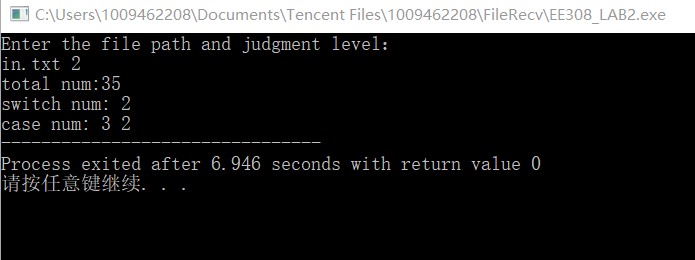# EE308_LAB2

The Link of Requirement of This Assignmenthttps://bbs.csdn.net/topics/600798588
The Aim of This Assignment1. To achieve a program function 2. Extract keywords of different levels from the C++ code files that are read in 3. learn the use of github
MU STU ID and FZU STU ID19103921_831902217

#### ✔Content

https://github.com/yyx0115/EE308_LAB2/tree/main

#### 📝PSP FORM

Personal Software Process stageEstimated Time（min）Actual Time(min)
Planning
Estimate3030
Development
Analysis100120
Design Spec60100
Design Review1515
Coding Standard3030
Design180200
Coding12001600
Code Review6060
Test100100
Reporting
Test Report3030
Size Measurement1515
Postmortem & Process Improvement Plan6060
Sum of Time18802360

#### 🌋Description of problem-solving ideas

1. Choose Language
• c++/c
• Python
• java
1. Review the knowledge of C/C++
we need to know the keywords:After last semester’s study, I acquired some knowledge about Java, C ++/ C. It’s a pity that I didn’t learn Python yet. After a summer vacation of “indulgence”, before starting this assignment, I need to review the “if-else” knowledge to wake up my memory

1. rough thinking

• Create a user input class

• a search class to find the keywords

• a class to calculate the number of keywords and the times of switches and cases

• use pointer function probably

• the number of if-else and if else if else

### 💫Code Description

1. create a file named “in.txt” include⤵:
#include <stdio.h>
int main(){
int i=1;
double j=0;
long f;
switch(i){
case 0:
break;
case 1:
break;
case 2:
break;
default:
break;
}
switch(i){
case 0:
break;
case 1:
break;
default:
break;
}
if(i<0){
if(i<-1){}
else{}
}
else if(i>0){
if (i>2){}
else if (i==2) {}
else if (i>1) {}
else {}
}
else{
if(j!=0){}
else{}
}
return 0;
}

1. The input⤵：

#include<bits/stdc++.h>
using namespace std;
map<string,int> mp;
string words={"auto","case","char","const","do",
"double","enum","extern","float","goto",
"int","long","register","short","signed","static",
"struct","typedef","union","unsigned","void","volatile","while"
,"break","continue","for","if","sizeof","switch","return","default","else"};

2. open and read the file⤵:

void getNum(char *s,int grade){
queue<int> q;
ifstream infile;
infile.open(s,ios::in);
if (!infile){
printf("file opened failure！！！");
return ;
}

1. To find the switch and case numbers⤵:
	string temp;
bool judgeswitch=false;
int cntcase;
while (!infile.eof()){
infile>>temp;
//cout<<temp<<endl;
for (int i=0;i<32;i++){
if (i<22){
if (temp==words[i]){
mp[temp]++;
if (temp=="case"&&judgeswitch)   cntcase++;
}
}
else{
if (temp.substr(0,words[i].size())==words[i]){
if (temp.size()==words[i].size()){
mp[words[i]]++;
if (words[i]=="switch"){
judgeswitch=true;
cntcase=0;
}
else if (words[i]=="default"){
judgeswitch=false;
q.push(cntcase);
}
}
else if (temp[words[i].size()]=='('||temp[words[i].size()]==';'||temp[words[i].size()]==':'
||temp[words[i].size()]=='{'){
mp[words[i]]++;
if (words[i]=="switch"){
judgeswitch=true;
cntcase=0;
}
else if (words[i]=="default"){
judgeswitch=false;
q.push(cntcase);
}
}
}
}
}
}

1. output and the main function⤵:
int total=0;
for (auto v:mp){
//output all the keywords
//cout<<v.first<<" : "<<v.second<<endl;
//calculate the sum
total+=v.second;
}
cout<<"total num:"<<total<<endl;
cout<<"switch num: "<<q.size()<<endl;
cout<<"case num: ";
while (!q.empty()){
cout<<q.front()<<" ";
q.pop();
}
}
}
int main(){
char url;
cout<<"Enter the file path and judgment level："<<endl;  // in.txt level 2
return 0;
}

1. The output⤵#### The entire code

#include<bits/stdc++.h>
using namespace std;
map<string,int> mp;
string words={"auto","case","char","const","do",
"double","enum","extern","float","goto",
"int","long","register","short","signed","static",
"struct","typedef","union","unsigned","void","volatile","while"
,"break","continue","for","if","sizeof","switch","return","default","else"};

queue<int> q;
ifstream infile;
infile.open(s,ios::in);
if (!infile){
printf("file opened failure！！！");
return ;
}
string temp;
bool judgeswitch=false;
int cntcase;
while (!infile.eof()){
infile>>temp;
//cout<<temp<<endl;
for (int i=0;i<32;i++){
if (i<22){
if (temp==words[i]){
mp[temp]++;
if (temp=="case"&&judgeswitch)   cntcase++;
}
}
else{
if (temp.substr(0,words[i].size())==words[i]){
if (temp.size()==words[i].size()){
mp[words[i]]++;
if (words[i]=="switch"){
judgeswitch=true;
cntcase=0;
}
else if (words[i]=="default"){
judgeswitch=false;
q.push(cntcase);
}
}
else if (temp[words[i].size()]=='('||temp[words[i].size()]==';'||temp[words[i].size()]==':'
||temp[words[i].size()]=='{'){
mp[words[i]]++;
if (words[i]=="switch"){
judgeswitch=true;
cntcase=0;
}
else if (words[i]=="default"){
judgeswitch=false;
q.push(cntcase);
}
}
}
}
}
}
int total=0;
for (auto v:mp){
//output all the keywords
//cout<<v.first<<" : "<<v.second<<endl;
//calculate the sum
total+=v.second;
}
cout<<"total num:"<<total<<endl;
cout<<"switch num: "<<q.size()<<endl;
cout<<"case num: ";
while (!q.empty()){
cout<<q.front()<<" ";
q.pop();
}
}
}
int main(){
char url;
cout<<"Enter the file path and judgment level："<<endl;  // in.txt level 2
return 0;
}



#### 🎄Summarize this assignment

• I gained new knowledge on how to use GitHub,created my own GitHub page. ♪(´▽｀)

• wrote code in the process of consolidating and reviewing the previous courses. After relearning, I found many deficiencies and did not grasp many details in place. ( •̀ ω •́ )y

• Learned how to draw a flow chart with markdown and plan in advance with PSP form, which greatly improved the efficiency

• I feel very guilty for not completing the last two levels of questions, and my ability still needs to be improved. `(>﹏<)′

• The knowledge points of file flow need to be further strengthened. The reason why we choose to use C + + is that the knowledge of Java is not proficient enough and needs to be further study.03-2702-11
02-15
03-10
03-21
09-2263
09-23123
03-22272
11-1412
11-23524点击重新获取扫码支付余额充值